# 555 timer internal schematic questions

jearls74
hi everyone, i am trying to build a very high current dc to ac inverter and i can't use a 555 timer IC because they can't source or sink but a maximum of 200mA, but i have found an internal schematic of the 555 timer IC online and have been studying it to see if i can build a very high current version by changing all the resistor values and calculating currents so i can choose the correct NPN and PNP transistors and i have run into a few things i can't figure out and need some help. I am attaching the schematic i found online for reference to my questions. the first question i have is are resistors R1, R2,and R3 in series or parallel? the next question is how does the comparators compare the threshold input voltage to the fixed reference voltage of 2/3 of Vcc in the voltage divider and the trigger input to the fixed reference voltage of 1/3 of Vcc in the voltage divider? when i trace the conventional current flow through the transistors based on their biasing, i find the current flowing through the right side doesn't interact with the current flowing on the left side except where the combine before going through R5 and going to ground in the upper comparator. how does the threshold input know that the discharge capacitor has reach the fixed reference voltage of 2/3 Vcc if the current and voltage from the threshold input side (+ve) isn't flowing into the 2/3 Vcc reference voltage side (-ve) or vice versa? the last question for now is what kind of transistor is Q19? its a PNP but it has an extra collector, does anyone know what kind connection that is? just to describe what I am trying to accomplish by building this, i want to drive the bases of a small string of NPN and PNP transistors with the square wave output, so I am tryig to build this oscillator capable of sourcing and sinking 5 or 6 amps so i can pull 100 to 150 amps of AC. any help is appreciated

#### Attachments

Last edited:

I have a better diagram in a Signetics Data book, so I have uploaded it.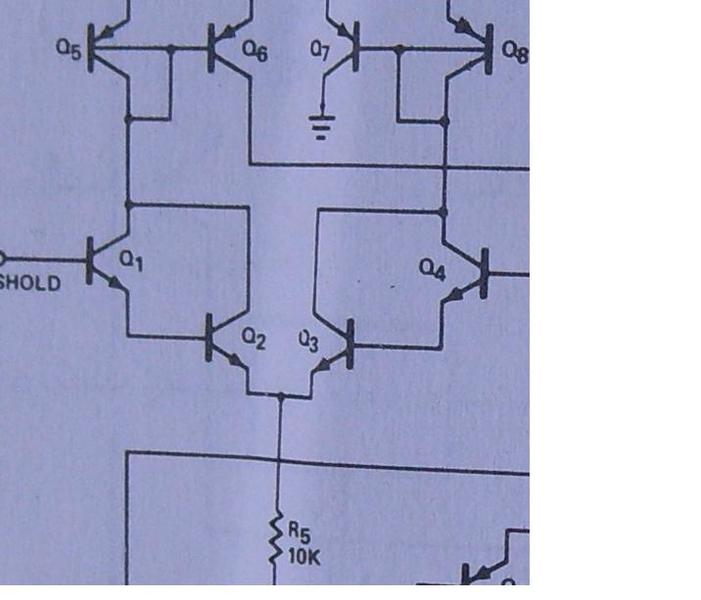If the base voltage of Q1 is higher than the reference (set by the current through Q3 in R3) then Q1 will turn on causing Q6 to turn on and send current from its collector to Q16.

Q19 is some oddball thing they created inside an IC. They can do stuff like that. You can't buy a discrete transistor like that.

150 amps is a lot of current. How are you planning to do that?

Last edited:
Bob S
I do not believe the 555 is capable of producing a true 50% duty cycle square wave. You should include a divide-by-2 JK (not D-type) flip flop somewhere in the circuit before the current amplification.
Bob S

Last edited:
jearls74
thanks for the information vk6kro, I am still a little confused on how the comparators compare the 2 different voltages? i have indicated conventional current flow on the schematic for the upper comparator and still can't figure out how the threshold input (+ve) knows that there is 8 volts on the reference input (-ve) as the threshold input monitors the external capacitor? is the voltages compared at the emitters of Q2 and Q3? and are the voltages compared at the emitters of Q11 and Q12 in the lower comparator? also, i can't figure out if R1 , R2 , and R3 are in series or parallel? would they be in parallel since the transistors are in parallel? also, on the transistor Q19, could i use a regular PNP transistor connected the way i have shown in the schematic so as to eliminate the extra collector it is showing connected to the base? any help is greatly appreciated.

#### Attachments

jearls74
thanks for the replies vk6kro and bob, the goal of this project is to build a mobile dc to ac inverter that is capable of supplying a very large current at 12 volts so i can step it down though a large transformer i am constructing. i want to take 12 volts at 100 amps or more and step it down to 6 volts at around 200 amps so i can generate a very large magnetic force for a small rail gun i want to build. I am just a hobbyist and not an electrical engineer or student. what i want to do is take a schematic i found that started my quest on this project and alter it to suit my needs. i have built the one in the schematic and it worked briefly but the 555 timer IC chip kept getting fried because it was being forced to sink more than 400 mA or more so I am trying to construct an oscillator by changing the internal components so i can source and sink 5 or 6 amps to feed the bases of 4 or 5 sets of PNP and NPN transistor in parallel, the filter the output through a few high capacitance capacitor and inductor filters before being stepped down in the transformer. on output from the transformer i will use 4 or 5 bridge rectifiers in parallel to convert the AC back into a DC and connect each rectifier to a high capacitance capacitor capable of storing 45 to 50 amps. these will be discharged all at once into the rails of the railgun to produce a tremendous magnetic force I am hoping. most of this is still just theory as i haven't put much into anything past figuring out the oscillator part of all this first.

#### Attachments

Bob S
Thanks for your last post. If the objective is to step the voltage down, maybe a better choice would be a switch-mode step-down converter, such as SEPIC or pulse width modulated. This will be more efficient. If you want to use a dc-ac and ac-dc at high power, there should not be any average dc current in any transformer windings, so you need a true 50% duty cycle square wave..
Bob S

In the following diagram,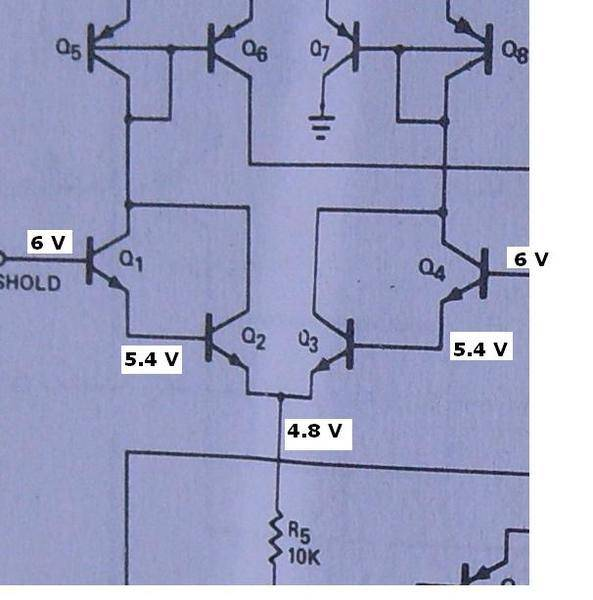Suppose the reference voltage (coming from the right on the base of Q4) was 6 volts.

This would produce a voltage of 5.4 volts on the base of Q3 and 4.8 volts on the emitter of Q3. This is a drop of 0.6 volts on each base emitter junction.

Now, for Q2 to conduct, there must be a voltage of at least 5.4 volts on its base (0.6 volts greater than 4.8 volts on its emitter) and likewise, there must be a voltage of more than 6 volts on the base of Q1 for it to conduct.

This is how the comparator works.

Notice that this 6 volts is very cleverly arranged to be the same as the reference voltage on Q4.

R1, R2, R3 and R4 are not in series or parallel with each other because there are other components connected to them. They can only be in parallel if they are directly connected across each other.

If you wanted a true square wave, the following circuit produces a good one. It needs the CMOS version of the 555, but these are readily available.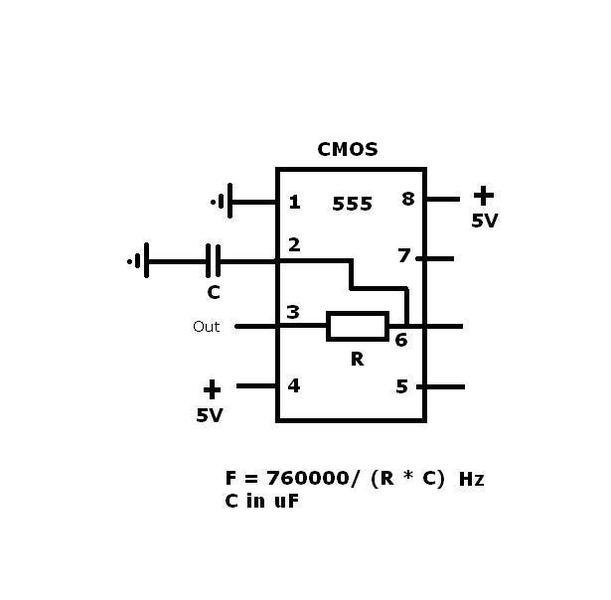jearls74
Thanks vk6kro your explanation explains a lot about the comparator operation. Are the arrows i have drawn on the schematic that show conventional current flow wrong? i don't understand how Q1 can turn on Q6? Doesnt current flowing out of the bases of Q5 and Q6 combine after the emitter of Q5 and flow down to the node after the emitter of Q1 where it combines and flows to the collector of Q2 ?

have a look at this: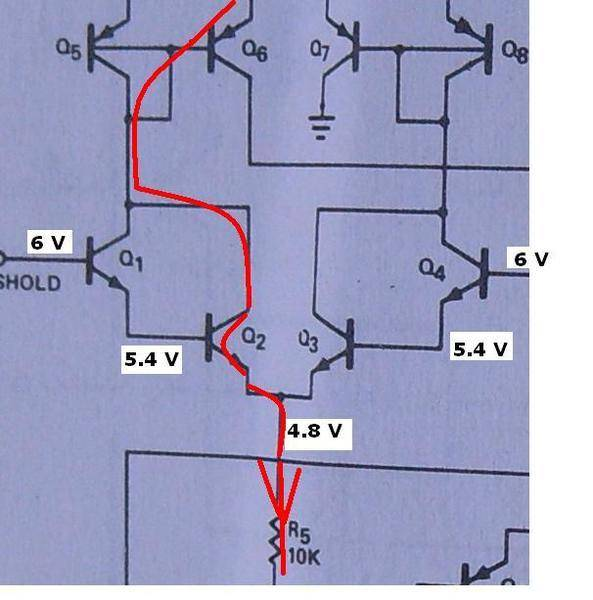For Q6 to turn on, it must have base current.
I have drawn a red line to show the path of the base current when the input voltage is greater than the threshold (6 volts in this case).

jearls74
Thanks for the answer vk6kro, the more time i spend looking at the schematics, the more confused i get. I understand the comparator part now thanks to you. Now I am trying to figure out the current path for high output stage and low output stage. I have drawn a blue line on the schematic for low output stage and a red line for the high output stage( what i think it is anyway). Are both comparators on at the same time? how can the lower comparator output turn on Q15 if there's no current flowing from Q6 to turn on the base of Q16 and flow into the collector of Q15? Does the current flowing out of the bases of Q9 and Q19 flow through R10 and diode Q18 into the collector of Q16? and which state is that happening in? I also have a resistor question, since R1, R2, R3 and R4 arent in series or parallel, would ohms law apply to each one seperately to calculate current through them? i could probably do a few calculation that might now be right and build this circuit, but i would really like to understand what's happening inside it in its two states. thanks for any help

#### Attachments

BenchTop
This is a passive voltage regulator circuit and is unable to increase current.

Doubling current output can't be done passively, so perhaps a voltage regulator is not the best path to get you from here to where you want to be.

The simplest solution to powering coils with a live supply may be simply a transformer, bridge rectifier, big caps.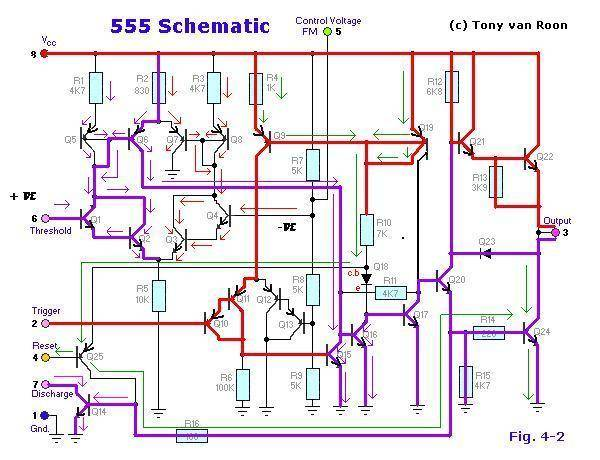I have added the arrows on the transistors so you can tell which are NPN or PNP.

The comparators are not on at the same time. The lower one comes on briefly if the capacitor voltage drops below 1/3 supply and the top one comes on if it goes above 2/3 supply.

But they have to trigger for long enough for the capacitor to charge or discharge. This is achieved with Q16 and Q17 which form an S-R flipflop. The vital component in this is the 4.7K resistor (R11) which holds Q16 conducting after the pulse from the top comparator turns it on.

This is arranged to turn the discharge pin on and off to discharge the capacitor when it is charged and to charge it when it is discharged.

Shorting out the base of Q16 by turning Q15 on resets this flipflop and stops the discharge cycle.

Last edited:
jearls74
Thanks for continuing to help me figure this out vk6kro, I am understanding more each time you help. I have another question after studying your last reply. Capacitor discharges to below 1/3 Vs (4 volts) and trigger pulse turns on Q15 which connects Q16's base to ground and turns off Q16, Q17, and Q20 which turns off the discharge transistor and allows capacitor to start charging? Here the question, what happens to current through R10 and diode Q18?does it not continue back into the base of Q17 which would keep Q20 on? and how is Q20 turned off so it doesn't continue to keep the base of Q20 on? i appreciate all your help.

Because the 4.7K resistor is holding Q16 fully on, it doesn't matter what the current in the diode does. It can't turn Q17 on because its base is almost grounded by the output of Q16.

and how is Q20 turned off so it doesn't continue to keep the base of Q20 on?

Not sure what that means.

Q15 starts the process of turning off the discharge cycle. Just follow it through. Each stage inverts the output of the stage before it.

jearls74
sorry about the typo vk6kro, i meant how do you turn off Q19 ? i was thinking if the base of Q19 was turned on and current was flowing from its collector, wouldn't it flow to the base of Q20 and keep it conducting?

sorry about the typo vk6kro, i meant how do you turn off Q19 ? i was thinking if the base of Q19 was turned on and current was flowing from its collector, wouldn't it flow to the base of Q20 and keep it conducting?

No, if Q17 is conducting, Q20's base is pulled low enough that there is no base current flowing.

jearls74
Thanks vk6kro, I am learning more with every reply, like i have said, I am just a hobbyist, everything i know i learned on the internet, I am tracing the paths like you said and ill post what i come up with but i don't understand what " Q20's base is pulled low enough that no base current will flow" can you explain what that is?

Ok.

Can you see that for Q24 or Q14 to turn on their base voltages must be at least 0.6 volts? And for Q20 to turn on its base must be 0.6 volts more than this? (Because its emitter is at 0.6 volts) So, it must have 1.2 volts on the base to turn on.

But if Q17 is turned on fully, it will have about 0.2 volts on its collector which is connected to the base of Q20. So Q20 cannot be turned on.

jearls74
I forgot to consider the turn on voltage for the transistors, I've got so focused on trying to to figure every path that current can flow through each transistor to complete the circuit. I guess my next question is about the transistor, I've notice on all the transistor calculator online I've used, thaton a NPN , the voltage on the emitter is the voltage through the base minus the .6 diode junction drop and on the PNP its the voltge on the collector minus the .6 volt diode drop, what happens to the voltage being input to the collectors? Thanks for all your time and knowledge.

A PNP transistor is exactly the same as a NPN except the polarity is reversed.

Last edited:
jearls74
are the transistors in saturation when they are on? like they are used as switches?

You would have to look at the circuitry for each transistor.

Generally, if they have an emitter resistor they are probably not in saturation.

If the emitter is just grounded and there is a small base bias resistor, they probably are being used as a switch.

jearls74
Ok, I am trying to calculate the currents and voltages of the transistors starting with Q5 but I am not really sure if my numbers are right because i used a formula for a NPN to calculate Q5 which is a PNP, so i altered the formula to account for the base current. Can somebody please look over my numbers to see if I am correct and if I am not, can you help get me on the right path? I plan on using very low value resistors so i can get maximum current flow, so i need to get the right numbers so i can purchase the correct transistors that can handle this much power. Anyway, here's what i came up with for Q5: (R1 is 4.7 ohms)

Ib= Ie * Beta
Ie= Ib * Beta - Ib
2.55A= Ib * 15 - Ib
2.55A= 14Ib
Ib= 2.55A/14
Ib= .182mA

I get .182mA leaving the base of Q5. Calculating Ic i get:

Ic= Ie - Ib
Ic= 2.55A - .182mA
Ic= 2.36A
My calculation for voltage is:

Vre= Ie * Re
Vre= 2.36A * 4.7Ohms
Vre= 11.09V
On this part i used a Tina-Ti simulation and since R1 Is a current limiting resistor, it doesn't drop the 11.09V, but drops .300 mV according to the simulation.

Power dissapation through the resistor is:

Pre = I2 * R
Pre = 2.55 I2 *4.7 Ohms
Pre = 30.56 Watts

Power dissapation through the transistor is:

Pq = I * E
Pq = 2.36A * 11.09V
Pq = 2.36A * 11.09 - 0.07 Vbe
Pq = 2.36A * 10.39V
Pq = 26.49 Watts

can someone please look over these numbers ? thanks

Mordy777
I agree with Bob. I do not believe the 555 is capable of producing a true 50% duty cycle square wave. You should include a divide-by-2 JK (not D-type) flip flop somewhere in the circuit before the current amplification.

http://www.rs232-converters.com/

jearls74
Thanks for the reply Mordy, the output duty cycle doesn't have to be a precise 50%, the timer calculation i have been using for calculating the duty cycle gives a 52% duty cycle with Ra=10 ohms Rb= 100 and capacitor of 100 uF and a 68 hz frequency.I will look into the divide by 2 JK flip-flop.